## RS Aggarwal Class 8 Solutions Chapter 1 Rational Numbers Ex 1B

These Solutions are part of RS Aggarwal Solutions Class 8. Here we have given RS Aggarwal Solutions Class 8 Chapter 1 Rational Numbers Ex 1B.

Other Exercises

Question 1.
Solution:
(i) $$\\ \frac { 1 }{ 3 }$$
Draw a line and take a point O on it.
Let it represent 0.
From O, set off unit OA, AB to right side of O, representing integers 1, 2 respectively.
Now, divide OA into 3 equal parts and take one part OR Which is $$\\ \frac { 1 }{ 3 }$$ as shown below on the line.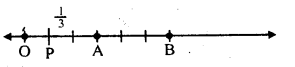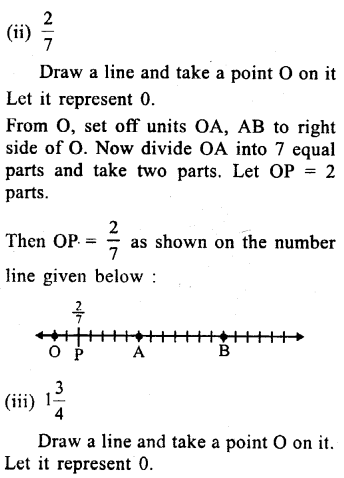Question 2.
Solution:
(i) $$\\ \frac { -1 }{ 3 }$$
Draw a line and take a point O on it.
Let it represent O.
Now, from O, take OA, AB to the left of O, representing integers – 1, – 2 respectively. Divide OA into three equal parts and take one part.
Then OP = $$\\ \frac { -1 }{ 3 }$$ which is shown on the number line as given below :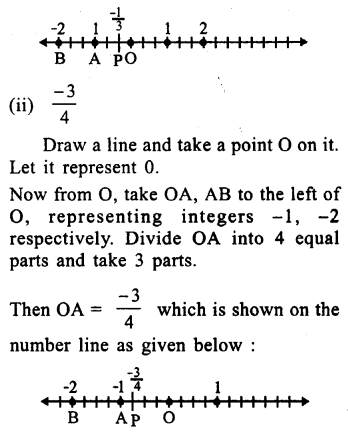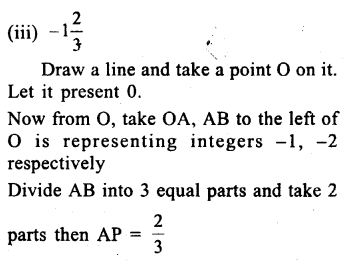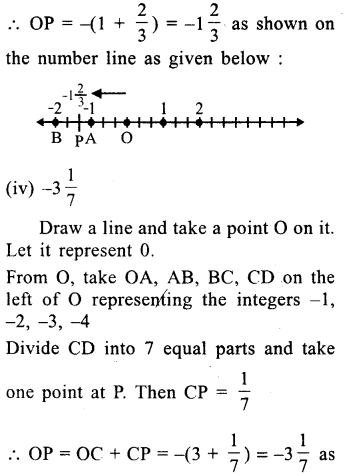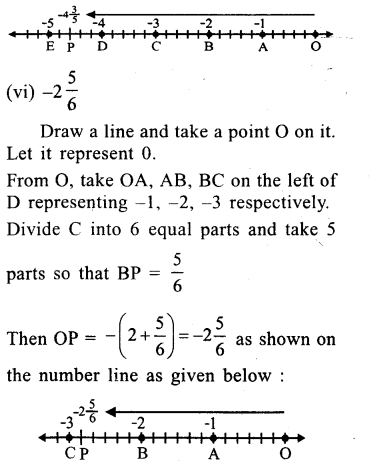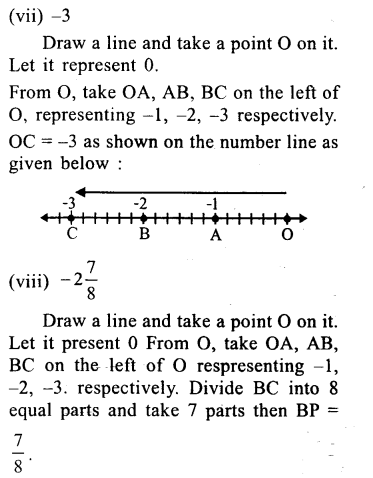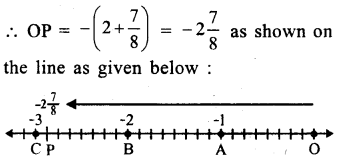Question 3.
Solution:
(i) True, as the numbers left of O are negative.
(ii) False, as the numbers right of O are positive and $$\\ \frac { -12 }{ 7 }$$ is negative.
(iii) True, as $$\\ \frac { 1 }{ 3 }$$ is positive and $$\\ \frac { -5 }{ 2 }$$ is negative.
(iv) False $$\\ \frac { -18 }{ -13 }$$ = $$\\ \frac { 18 }{ 13 }$$ which is positive and positive numbers lie on the right of O.

Hope given RS Aggarwal Solutions Class 8 Chapter 1 Rational Numbers Ex 1B are helpful to complete your math homework.

If you have any doubts, please comment below. Learn Insta try to provide online math tutoring for you.